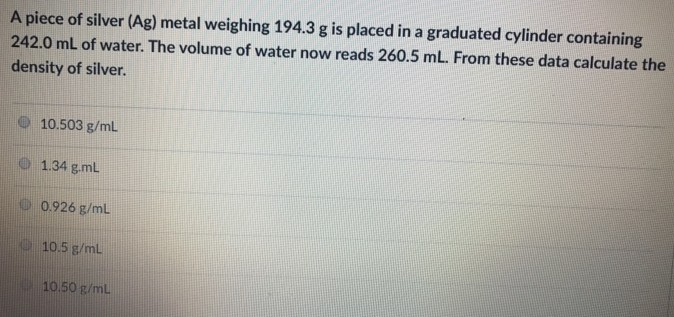# Problem: A piece of silver (Ag) metal weighing 194.3 g is placed in a graduated cylinder containing 242.0 mL of water. The volume of water now reads 260.5 mL. From these data calculate the density of silver. a. 10.503 g/mL b. 1.34 g/mL c. 0.926 g/mL d. 10.5 g/mLe. 10.50 g/mL

###### FREE Expert Solution
91% (419 ratings)###### Problem Details

A piece of silver (Ag) metal weighing 194.3 g is placed in a graduated cylinder containing 242.0 mL of water. The volume of water now reads 260.5 mL. From these data calculate the density of silver.

a. 10.503 g/mL

b. 1.34 g/mL

c. 0.926 g/mL

d. 10.5 g/mL

e. 10.50 g/mL